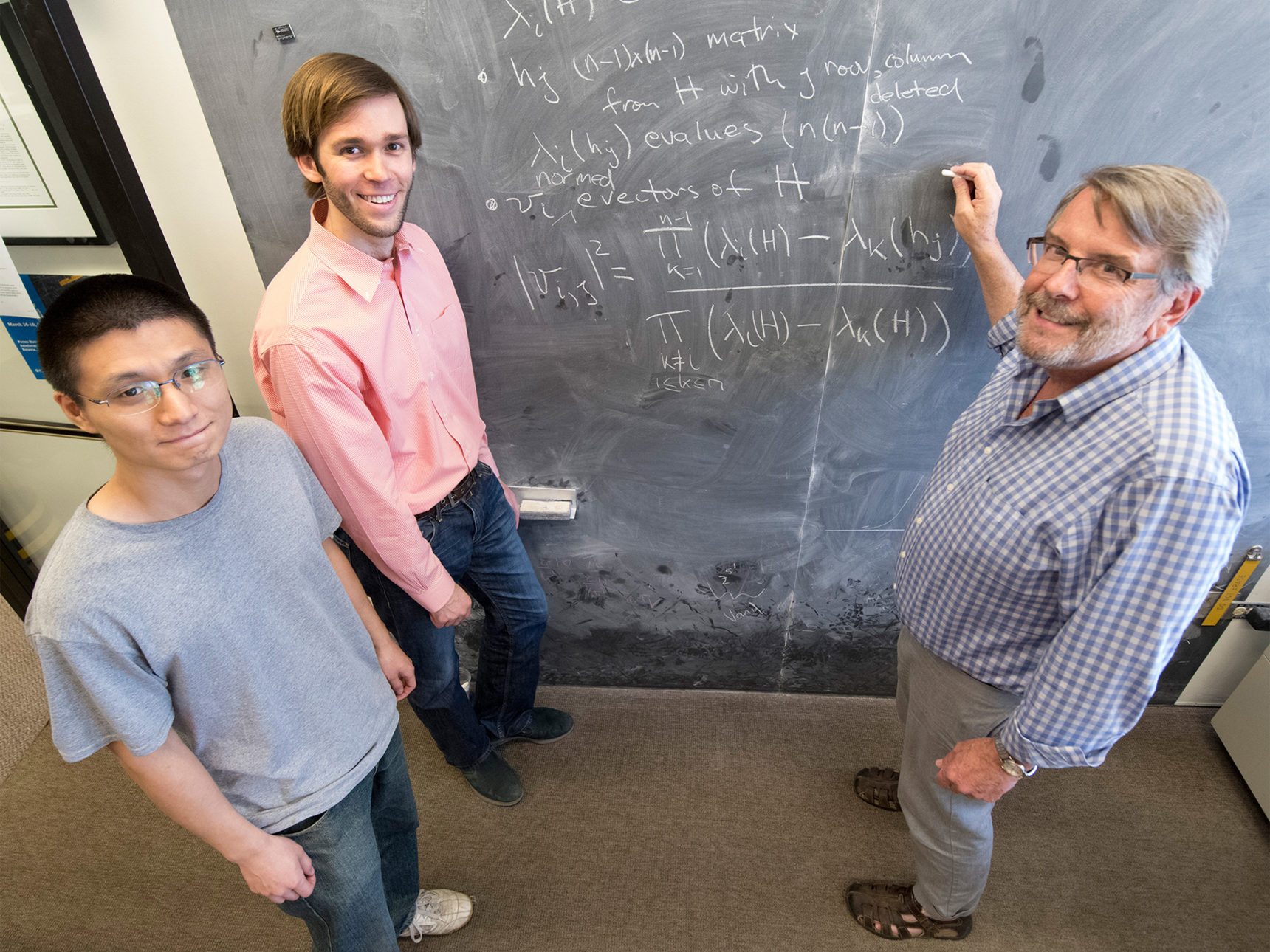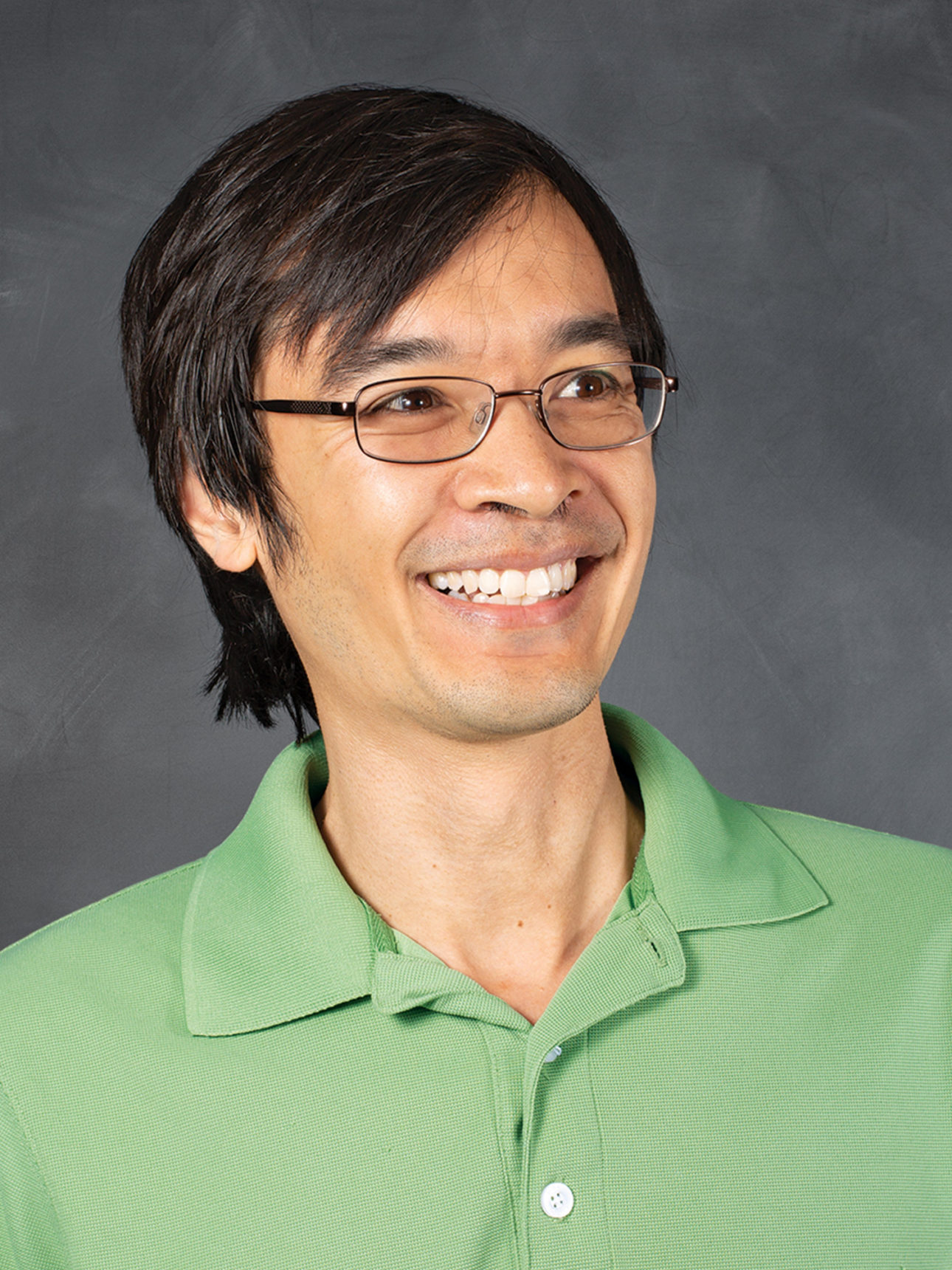# Neutrinos Lead to Unexpected Discovery in Basic Math

## Three physicists wanted to calculate how neutrinos change. They ended up discovering an unexpected relationship between some of the most ubiquitous objects in math.

fter breakfast one morning in August, the mathematician Terence Tao opened an email from three physicists he didn’t know. The trio explained that they’d stumbled across a simple formula that, if true, established an unexpected relationship between some of the most basic and important objects in linear algebra.

The formula “looked too good to be true,” said Tao, who is a professor at the University of California, Los Angeles, a Fields medalist, and one of the world’s leading mathematicians. “Something this short and simple — it should have been in textbooks already,” he said. “So my first thought was, no, this can’t be true.”

Then he thought about it some more.

The physicists — Stephen Parke of Fermi National Accelerator Laboratory, Xining Zhang of the University of Chicago and Peter Denton of Brookhaven National Laboratory — had arrived at the mathematical identity about two months earlier while grappling with the strange behavior of particles called neutrinos.

They’d noticed that hard-to-compute terms called “eigenvectors,” describing, in this case, the ways that neutrinos propagate through matter, were equal to combinations of terms called “eigenvalues,” which are far easier to compute. Moreover, they realized that the relationship between eigenvectors and eigenvalues — ubiquitous objects in math, physics and engineering that have been studied since the 18th century — seemed to hold more generally.

Although the physicists could hardly believe they’d discovered a new fact about such bedrock math, they couldn’t find the relationship in any books or papers. So they took a chance and contacted Tao, despite a note on his website warning against such entreaties.

“To our surprise, he replied in under two hours saying he’d never seen this before,” Parke said. Tao’s reply also included three independent proofs of the identity.

A week and a half later, the physicists and Tao, whom Parke called “a fire hose of mathematics,” posted a paper online reporting the new formula. Their paper is now under review by Communications in Mathematical Physics. In a separate paper submitted to the Journal of High Energy Physics, Denton, Parke and Zhang used the formula to streamline the equations governing neutrinos.From left: Xining Zhang, Peter Denton and Stephen Parke in front of the formula they discovered.

Experts say that more applications might arise, since so many problems involve calculating eigenvectors and eigenvalues. “This is of really broad applicability,” said John Beacom, a particle physicist at Ohio State University. “Who knows what doors it will open.”

Pure mathematicians feel similarly. “This is certainly both surprising and interesting,” said Van Vu, a mathematician at Yale University. “I did not suspect that one can compute eigenvectors using only information about eigenvalues.”

Vu and Tao proved a somewhat related identity together in 2009 (which is why Denton, Parke and Zhang thought to contact Tao to begin with), but the new formula does not obviously follow from the older one. And although a similar formula happened to appear in a mathematics paper in May of this year, the authors of that paper did not make the link to eigenvectors and eigenvalues.

In a way, it’s not surprising that a new insight into centuries-old mathematical objects came from physicists. Nature has inspired mathematical thinking ever since humans started counting on 10 fingers. “For math to thrive, it has to connect to nature,” Vu said. “There is no other way.”

## Transformer Tricks

Eigenvectors and eigenvalues are ubiquitous because they characterize linear transformations: operations that stretch, squeeze, rotate or otherwise change all parts of an object in the same way. These transformations are represented by rectangular arrays of numbers called matrices. One matrix might rotate an object by 90 degrees; another might flip it upside down and shrink it in half.

Matrices do this by changing an object’s “vectors” — mathematical arrows that point to each physical location in an object. A matrix’s eigenvectors — “own vectors” in German — are those vectors that stay aligned in the same direction when the matrix is applied. Take, for example, the matrix that rotates things by 90 degrees around the x-axis: The eigenvectors lie along the x-axis itself, since points falling along this line don’t rotate, even as everything rotates around them.

A related matrix might rotate objects around the x-axis and also shrink them in half. How much a matrix stretches or squeezes its eigenvectors is given by the corresponding eigenvalue — in this case, ½. (If an eigenvector doesn’t change at all, the eigenvalue is 1.)Eigenvectors and eigenvalues are independent, and normally they must be calculated separately starting from the rows and columns of the matrix itself. College students learn how to do this for simple matrices. But the new formula differs from existing methods. “What is remarkable about this identity is that at no point do you ever actually need to know any of the entries of the matrix to work out anything,” said Tao.

The identity applies to “Hermitian” matrices, which transform eigenvectors by real amounts (as opposed to those that involve imaginary numbers), and which thus apply in real-world situations. The formula expresses each eigenvector of a Hermitian matrix in terms of the matrix’s eigenvalues and those of the “minor matrix,” a smaller matrix formed by deleting a row and column of the original one.

The formula makes sense in hindsight, Tao said, because the eigenvalues of the minor matrix encode hidden information. But “it certainly was not something that I, for instance, would have thought about.”

It’s unusual in mathematics for a tool to appear that’s not already associated with a problem, he said. But he thinks the relationship between eigenvectors and eigenvalues is bound to matter. “It’s so pretty that I’m sure it will have some use in the near future,” he said. “Right now, we just have one application.”

## Shape-Shifting Particles

That application would be neutrinos: the oddest, least understood, most reclusive of the known fundamental particles. Neutrinos pass through each of our bodies by the trillion each second, but because they barely register, many of their properties remain unknown.

Intriguingly, theory suggests that differences in the behavior of neutrinos and antineutrinos could be what allows matter to dominate over antimatter in the universe. If these opposites had arisen in equal amounts in the Big Bang, they would have mutually annihilated, yielding a cosmos empty of everything except light. A distinction between neutrinos and antineutrinos could be what allowed the all-important surplus of matter to accrue. “If they act differently, that will give us some hint as to why the universe is filled with matter,” said Deborah Harris, a physicist at York University and Fermilab who works on a neutrino experiment called DUNE (for Deep Underground Neutrino Experiment) aimed at measuring such differences.

The experiment, which will measure neutrinos shot from Fermilab in Illinois to an underground detector 1,300 kilometers away in South Dakota, makes use of the fact that neutrinos come in one of three possible “flavors” — electron, muon or tau. But each neutrino flavor is a quantum mechanical mixture, and neutrinos oscillate between flavors on the fly. As a neutrino from Fermilab travels along, its mixture changes, so that a muon neutrino might morph into an electron neutrino or a tau neutrino.

An egregiously complicated three-by-three matrix describes these oscillations. From the eigenvectors and eigenvalues, physicists can calculate an expression for the likelihood that a muon neutrino will oscillate into an electron neutrino by the time it reaches South Dakota. They can also calculate an expression for the probability that a muon antineutrino will become an electron antineutrino.

Both expressions contain an unknown: the “CP violating phase,” which says how much the oscillatory patterns of neutrinos and antineutrinos differ. By measuring and comparing the actual oscillation rates, DUNE scientists can solve for that unknown. If the CP violating phase is large enough, this will help explain why the universe is filled with matter.

As if the calculations weren’t hard enough already, a bizarre effect first identified by the physicist Lincoln Wolfenstein in 1978 makes the neutrino matrix even more nightmarish. Neutrinos seldom interact with matter in the usual sense, but Wolfenstein realized that passing through matter rather than empty space nevertheless changes the way neutrinos propagate. As an electron neutrino zooms through matter, it will occasionally interact with an electron in an atom, effectively swapping places with it: The electron neutrino transforms into an electron and vice versa.

Such swaps introduce a new term in the matrix affecting electron neutrinos, which tremendously complicates the math. It’s this “Wolfenstein matter effect” that pushed Parke, Zhang and Denton to look for a way to simplify the calculations.

The expressions for the eigenvalues are simpler than those of eigenvectors, so Parke, Zhang and Denton started there. Previously, they had developed a new method to closely approximate the eigenvalues. With these in hand, they noticed that the long eigenvector expressions seen in previous works were equal to combinations of those eigenvalues. Putting the two together, “you can calculate neutrino oscillations in matter fast and simply,” Zhang said.

As for how they spotted the pattern that suggested the formula, the physicists aren’t sure. Parke said they simply noticed instances of the pattern and generalized. He admits to being good at solving puzzles. In fact, he is credited with co-discovering another important pattern in 1986 that has streamlined particle physics calculations and inspired discoveries ever since.

Still, the fact that the strange behavior of neutrinos could lead to new insights about matrices came as a shock. “People have been solving problems with linear algebra for a very, very long time,” Parke said. “I’m expecting someday to get an email from somebody that says, ‘If you look at this obscure paper by [the 19th-century mathematician] Cauchy, in the third appendix in a footnote, it’s there.’”

## A Minor Difference

In fact, a similar formula did already exist, but it had gone unnoticed because it was in disguise.

In September, Tao got another out-of-the-blue email, this time from Jiyuan Zhang, a mathematics graduate student at the University of Melbourne in Australia. Zhang pointed to an equivalent formula in a paper he’d co-authored with his adviser, Peter Forrester, back in May, before Tao and the physicists’ papers appeared. Zhang and Forrester were working in an area of pure mathematics called random matrix theory. They had applied the formula in their study of the randomized Horn problem — a problem connected to one that Tao and a colleague solved in 1999.

In an email to Quanta, Forrester explained that the formula first appeared in yet another form in a 2001 paper by Yuliy Baryshnikov, a mathematician now at the University of Illinois, Urbana-Champaign, whose work Forrester and Zhang had built on. These mathematicians hadn’t described the objects in their identity as eigenvectors, but rather as terms for calculating eigenvalues of certain minor matrices that arose in their problem.Forrester called the formula in his and Zhang’s paper “identical” to that of Tao and the physicists. Tao called the formulas “almost identical,” related to each other like halves of the rabbit-duck illusion. “Some people were looking only for rabbits, and other people were looking only for ducks,” he said.

Denton, in an email, deemed the preexisting formula “close to our result, but not exactly on the bull’s-eye.” He added, “In light of the importance of eigenvectors for many applications, we still think that our result is distinct enough to be considered new.”

It’s perhaps not so strange that there should be a sudden flurry of activity in a single summer after so many centuries. “There’s a lot of simultaneous discovery in mathematics,” Tao said. “Somehow the results are almost in the air. And people are just beginning to look in the right places.”